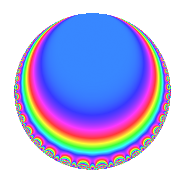# Properties

 Label 448.2.f.bLevel 448 Weight 2 Character orbit 448.f Analytic conductor 3.577 Analytic rank 0 Dimension 2 CM disc. -7 Inner twists 4

# Related objects

## Newspace parameters

 Level: $$N$$ = $$448 = 2^{6} \cdot 7$$ Weight: $$k$$ = $$2$$ Character orbit: $$[\chi]$$ = 448.f (of order $$2$$ and degree $$1$$)

## Newform invariants

 Self dual: No Analytic conductor: $$3.57729801055$$ Analytic rank: $$0$$ Dimension: $$2$$ Coefficient field: $$\Q(\sqrt{-7})$$ Coefficient ring: $$\Z[a_1, \ldots, a_{7}]$$ Coefficient ring index: $$2$$ Sato-Tate group: $\mathrm{U}(1)[D_{2}]$

## $q$-expansion

Coefficients of the $$q$$-expansion are expressed in terms of $$\beta = \sqrt{-7}$$. We also show the integral $$q$$-expansion of the trace form.

 $$f(q)$$ $$=$$ $$q -\beta q^{7} -3 q^{9} +O(q^{10})$$ $$q -\beta q^{7} -3 q^{9} -2 \beta q^{11} -2 \beta q^{23} + 5 q^{25} + 2 q^{29} -6 q^{37} -2 \beta q^{43} -7 q^{49} + 10 q^{53} + 3 \beta q^{63} + 6 \beta q^{67} -2 \beta q^{71} -14 q^{77} + 6 \beta q^{79} + 9 q^{81} + 6 \beta q^{99} +O(q^{100})$$ $$\operatorname{Tr}(f)(q)$$ $$=$$ $$2q - 6q^{9} + O(q^{10})$$ $$2q - 6q^{9} + 10q^{25} + 4q^{29} - 12q^{37} - 14q^{49} + 20q^{53} - 28q^{77} + 18q^{81} + O(q^{100})$$

## Character Values

We give the values of $$\chi$$ on generators for $$\left(\mathbb{Z}/448\mathbb{Z}\right)^\times$$.

 $$n$$ $$127$$ $$129$$ $$197$$ $$\chi(n)$$ $$-1$$ $$-1$$ $$1$$

## Embeddings

For each embedding $$\iota_m$$ of the coefficient field, the values $$\iota_m(a_n)$$ are shown below.

For more information on an embedded modular form you can click on its label.

Label $$\iota_m(\nu)$$ $$a_{2}$$ $$a_{3}$$ $$a_{4}$$ $$a_{5}$$ $$a_{6}$$ $$a_{7}$$ $$a_{8}$$ $$a_{9}$$ $$a_{10}$$
447.1
 0.5 + 1.32288i 0.5 − 1.32288i
0 0 0 0 0 2.64575i 0 −3.00000 0
447.2 0 0 0 0 0 2.64575i 0 −3.00000 0
 $$n$$: e.g. 2-40 or 990-1000 Significant digits: Format: Complex embeddings Normalized embeddings Satake parameters Satake angles

## Inner twists

Char. orbit Parity Mult. Self Twist Proved
1.a Even 1 trivial yes
7.b Odd 1 CM by $$\Q(\sqrt{-7})$$ yes
4.b Odd 1 yes
28.d Even 1 yes

## Hecke kernels

This newform can be constructed as the kernel of the linear operator $$T_{3}$$ acting on $$S_{2}^{\mathrm{new}}(448, [\chi])$$.